# Electronics and Communication Engineering - Exam Questions Papers

41.

A junction FET, can be used as a voltage variable resistor

 A. at pinch-off condition B. beyond pinch-off voltage C. well below pinch-off condition D. for any value of VDS

Answer: Option C

Explanation:

No answer description available for this question. Let us discuss.

42.

A fully charged mobile phone with a 12 V battery is good for a 20 minute talktime. Assume that, during the talk time, the battery delivers a constant current of 2 A and its voltage drops linearly from 12 V to 10 V as shown in the figure. How much energy does the battery deliver during this talk time?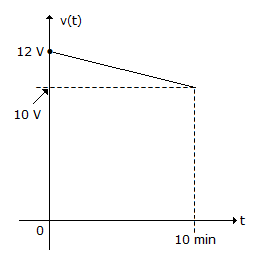A. 220 J B. 12 kJ C. 13.2 kJ D. 14.4 kJ

Answer: Option C

Explanation:

No answer description available for this question. Let us discuss.

43.

In a material, the Fermi level is located between the centre of the forbidden band and the conduction band. Then what is that material?

 A. A p-type semiconductor B. An n-type semiconductor C. An intrinsic semiconductor D. An insulator

Answer: Option B

Explanation:

No answer description available for this question. Let us discuss.

44.

Consider an SSB signal when the modulating signal is x(t). Let x(t) represents the complex conjugate of x(t) and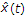is its Hilbert transform. The envelope of SSB signal is:

 A.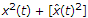B.C. x(t) D.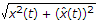Answer: Option D

Explanation:

No answer description available for this question. Let us discuss.

45.

Consider two independent random variables x and y with identical distributions. The variables X and Y take 0, 1 and2 with probabilities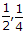and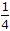respectively. What is the conditional probability P(X + Y = 2| X - Y = 0)?

 A. 0 B.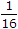C.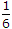D. 1

Answer: Option C

Explanation:

No answer description available for this question. Let us discuss.

#### Current Affairs 2021

Interview Questions and Answers